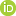This is a Preprint and has not been peer reviewed.

##### Authors

Denise Degen, Karen Veroy, Florian Wellmann

##### Abstract

One of the biggest challenges in Computational Geosciences is finding ways of efficiently simulating high-dimensional problems. In this paper, we demonstrate how the RB method can be gainfully exploited to solve problems in the Geosciences. The reduced basis method constructs low-dimensional approximations to (high-dimensional) solutions of parametrized partial differential equations. In contrast to other widely used geoscientific reduction techniques, the reduced basis method reduces the Galerkin approximation space, and not the physical space and is consequently much less restrictive. Another advantage of the method is that for the problems considered in this paper, the method provides a bound to the error in the reduced order approximation, thus permitting an objective evaluation of the approximation quality. Using a geothermal conduction problem we demonstrate that depending on the model we obtain a maximum speed-up of three orders of magnitude with an approximation error that is very small in comparison to typical measurement errors. This significant reduction of the cost of the forward simulation allows performing uncertainty quantification, inversions, and parameter studies for larger and more complex models than currently possible.

##### DOI

https://doi.org/10.31223/osf.io/neh9j

##### Subjects

Applied Mathematics, Earth Sciences, Numerical Analysis and Computation, Partial Differential Equations, Physical Sciences and Mathematics

##### Keywords

Finite element method, reduced basis method, Geothermal Conduction, Model Order Reduction, MOOSE Framework

##### Dates

Published: 2019-06-28 14:59